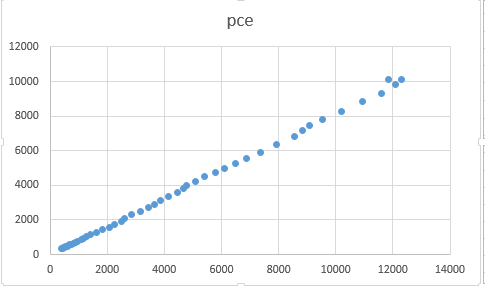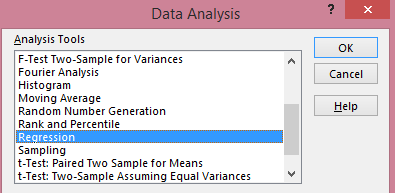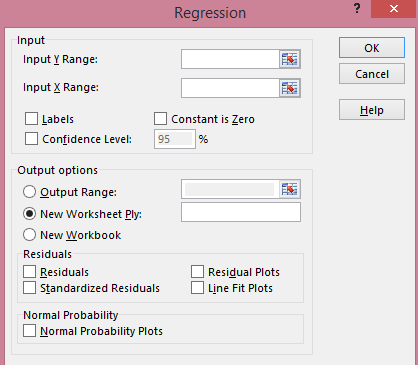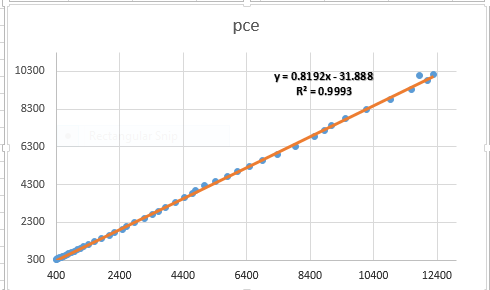## How to Interpret Regression Output in Excel

The dissertation buzz is on and students are doing everything possible to meet up with the deadline. This is the current atmosphere in tertiary institutions, at least for those with undisrupted academic calendar J. The students are in different stages of their project, as it is commonly called. Some are yet to wrap up their chapter one which gives the “study background” and the framing of research hypotheses, objectives and questions. Some have moved on to chapter two reviewing relevant literature related to their scope of study. Others have gone further in developing both the theoretical and empirical frameworks for chapter three, but not without the usual teething lags…but they’ll get around it, somehow J. A handful have even done better by progressing to chapter four attempting to analyse their data.

Since, chapters one to three are relative to each students’ scope of research, however, a regression output is common to all (although actual outcomes differ). It is based on this that I decided to do a tutorial in explaining the basic features in a regression output. Likewise, this write-up is in response to requests received from readers on (1) what some specific figures in a regression output are and (2) how to interpret their results. Let me state here that regardless of the analytical software whether Stata, EViews, SPSS, R, Python, Excel etc. what you obtain in a regression output is common to all analytical packages (except where slight variations occur).

For instance, in undertaking an ordinary least squares (OLS) estimation using any of these applications, the regression output will churn out the ANOVA (analysis of variance) table, F-statistic, R-squared, prob-values, coefficient, standard error, t-statistic, degrees of freedom, 95% confidence interval and so on. These are the features of a regression output. However, the issue is: what do these mean and how can they be interpreted and related to a research.

Hence, the essence of this tutorial is to teach students the relevance of these features and how to interpret their results. I will be using Excel analytical package to explain a regression output, but you can practise along using any analytical package of your choice. (See tutorial for Stata and EViews users).

An Example: Use Gujarati and Porter Table7_12.dta or Table7_12.xlsx dataset
Note: I will not be discussing stationarity or cointegration analysis in this tutorial (that will come later on). Since the issue on how to understand the features of a regression output and interpret results, I will just be doing a simple linear regression analysis (a bi-variate analysis) with only one explanatory variable.

The dataset is on the United States from 1960 to 2009 (50 years data). The outcome variable is consumption expenditure (pce) and the explanatory variable is income (income).

Before you begin, ensure that the DATA ANALYSIS Add-in is in your tool bar because without it, you cannot perform any regression analysis. To obtain it follow this guide:
File >> Options >> Add-ins >> Excel Options dialog box opens
Under Active Application Add-ins, choose Analysis ToolPak
In the Manage section, choose Excel Add-ins
Click Go, then OK

If it is correctly done, you should see this:Excel Add-in Dialog Box Source: CrunchEconometrix

Here is the data in excel format:

Third step: Visualise the relationship between the variables
Before analysing the data, it is good to always graph the dependent and key explanatory variable (using a scatter plot) in order to observe the pattern between them. This gives you what to expect in your actual analysis. Here’s the procedure:
1.    Highlight the 2 columns that contain the variables
2.    Go to Insert >> Charts >> ScatterExcel - Scatter plot of pce and income Source: CrunchEconometrix

The graph indicates a positive relationship between the two variables. This seems plausible because the higher your income, the higher will be your consumption, except you are very frugalJ.

The graph can be formatted by adding a trend line (see video on how to do this). In Excel, adding a trendline also gives you the linear prediction:

As can be seen from the second graph, we have the linear prediction for pce and the R2.

Fourth step: The scientific investigation
Now we want to scientifically investigate the relationship between pce and income. To do this, go to Data >> Data Analysis (dialogue box opens) >> Regression >> OK.Excel - Data Analysis Dialogue Box Source: Crunch Econometrix
Once you click OK, the Regression dialogue box opens:Excel - Regression Dialogue Box Source: CrunchEconometrix

·      Put data range for pce under Input Y Range
·      Put data range for income under Input X Range
·      Check label box
·      Check Confidence Level box
·      Check Output range
·      Click OK
(You have simply told Excel to regress the dependent variable, pce, on the explanatory variable, income), and the output is shown as:

Fifth step: The features of a regression output
The Excel output gives the Regression Statistics and the ANOVA table. So what do these figures mean? I will explain each feature in turns.

Under “Regression Statistics”:
R-squared: gives the variation in pce that is explained by income. The higher the R2, the better the model and the more predictive power the variables have. Although, an R2 that equals 1 will elicit some suspicion. The R is actually the correlation coefficient between the 2 variables. This implies that
= the correlation coefficient.

Standard Error: this is the standard error of the regression

Observations: the data span is from 1960 to 2009 = 50 years

Under “ANOVA” (analysis of variance):
Source: there are two sources of variation on the dependent variable, pce. Those explained by the regression (i.e, the Model) and those due to randomness (Residuals)

df: this is degree of freedom calculated as k-1 (for the model) and n-k (for the residuals)

SS: implies sum of squared residuals for the Regression (explained variation in pce) and Residuals (unexplained variation in pce). After doing the regression analysis, all the points on pce do not fall on the predicted line. Those points outside the line are known as residuals. Those that can be explained by the regression are known as Explained Sum of Squares (ESS) while those that are due to random forces, which are outside the model are known as Residual Sum of Squares (RSS).Excel - Predicted Value of pce Source: CrunchEconomterix
As observed from the graph, all the points do not fall on the predicted line. Some lie above, while some are beneath the line. These are all the residuals (in order words, the remnants obtained after the regression analysis). If the predicted line falls above a point, it means that pce is over-predicted (that is, pce – pcehat is negative) and if it is beneath a point, it implies that pce is under-predicted (that is, pce – pcehat is positive). The sum and mean of the residuals equals zero.

MS: implies mean sum of squared residuals obtained by dividing SS by df i.e. SS/df

F: captures whether the explanatory variable, income is significant in explaining the outcome variable, pce. The higher the F-stat, the better for the model.

Significance F: this is the probability value that indicates the statistical significance of the F ratio. A significance-value that is less than 0.05 is often preferred.

Coefficient: this is the slope coefficient. The estimate for income. The sign of the coefficient also tells you the direction of the relationship. A positive (negative) sign implies a positive (negative) relationship.

Intercept: this is the hypothetical outcome on pce if income is zero. It is also the intercept for the model.

Standard error: this is the standard deviation for the coefficient. That is, since you are not so sure about the exact value for income, there will be some variation in the prediction for the coefficient. Therefore, the standard error shows how much deviation occurs from predicting the slope coefficient estimate.

t-stat: this measures the number of standard errors that the coefficient is from zero. It is obtained by: coeff/std. error. A t-stat above 2 is sufficient evidence against the null hypothesis

P-value: there are several interpretations for this. (1) it is smallest evidence required to reject the null hypothesis, (2) it is the probability that one would have obtained the slope coefficient value from the data if the actual slope coefficient is zero, (3) the p-value looks up the t-stat table using the degree of freedom (df) to show the number of standard errors the coefficient is from zero, (4) tells whether the relationship is significant or not.

So, if the p-value is 0.3, then it means that you are only 70% (that is, (100-30)% ) confident that the slope coefficient is non-zero. This is not good enough. This is because a very low p-value gives a higher level of confidence in rejecting the null hypothesis. Hence, a p-value of 0.02, implies that you are 98% (that is, (100 - 2)% ) confident that the slope coefficient is non-zero which is more re-assuring! J.

Lower and Upper 95%: these are the confidence intervals. If the coefficient is significant, this interval will contain that slope coefficient but it will not, if otherwise.

Assignment:
Use Gujarati and Porter Table7_12.dta or Table7_12.xlsx dataset.
(1)  With pce as the dependent variable and gdpi as the explanatory variable, plot the graph of pce and gdpi, what do you observe?
(2)  Run your regression. Can you interpret the table and the features?
(3)  Plot the predicted line. What are your observations?

I have taken you through the basic features of a regression output using Excel data analysis software on ordinary least squares (OLS) model in a simple linear regression. So, you now have the basic idea of what the F-stat, t-stat, df, SS, MS, prob>F, p>|t|, confidence interval, R2, coefficient, standard error stand for.

[Watch video on "How to interpret regression output in Excel"]

Practice the assignment and if you still have further questions, kindly post them below....